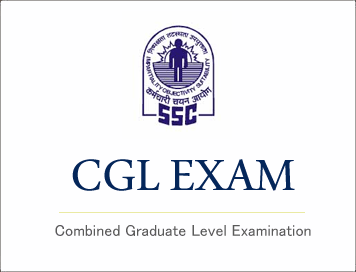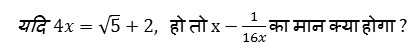# SSC CGL (Tier - 1) Online Exam Paper - 2016 "held on 09 September 2016" Morning Shift (Quantitative Aptitude)## SSC CGL (Tier - 1) Online Exam Paper - 2016 "held on 09 September 2016" Morning Shift (Quantitative Aptitude)

EXAM DATE : 9-September-2016
EXAM START TIME :
10:00:00
EXAM NAME :
SSC Examination 2016

Question 51.A & B together finish a job in 24 days, while A, B & C together can finish the same job in 8 days. C alone will finish the job in

Options:

1) 12 days
2) 14 days
3) 16 days
4) 24 days

Correct Answer: 12 days

Question 52.Area of the circle inscribed in a square of diagonal 6√2 cm (in sq cm) is

Options:

1) 9 Π
2) 6 Π
3) 3 Π
49√2Π

Correct Answer: 9 Π

Question 53.The original price of a TV set is Rs. 6,000. If the price is discounted by 20% and then raised by 10% for service contract, the price charged by the shopkeeper is

Options:

1) Rs. 5400
2) Rs. 5280
3) Rs. 5100
4) Rs.4200

Correct Answer: Rs. 5280

Question 54.A certain sum of money was divided between A, B and C in the ratio 5:6:9. If A received Rs. 450 the sum divided was

Options:

1) 2000
2) 1800
3) 2250
4) 1000

Question 55.By selling a bag at Rs. 230, profit of 15% is made. The selling price of the bag, when it is sold at 20% profit would be

Options:

1) Rs.250
2) Rs.205
3) Rs.240
4) Rs.200

Question 56.The weights of two iron balls are 3.5 kg and 7.5 kg. What is the percentage weight of the 1st ball with respect to 2nd ball.Question 57.A Bus travels at the speed of 36 km/hr, then the distance covered by it in one second is

Options:

1) 10 m
2) 15 m
3) 12.5 m
4) 13.5 m

Correct Answer: 10 m

Question 58.Options:

1) (a+b)/(a-b)
2) -1
3) 2ab
4) 1

## Study Kit for SSC CGL EXAM

Question 59.The value of (1 - √2) + (√2 - √3) + (√3 - √4) + ..... + (√15 - √16) is

Options:

1) 0
2) 1
3) -3
4) 4

Question 60.∆ABC and ∆DEF are two similar triangles and the perimeter of ∆ABC and ∆DEF are 30 cm and 18 cm respectively. If length of DE = 36 cm, then length of AB is

Options:

1) 60 cm
2) 40 cm
3) 45 cm
4) 50 cm

Correct Answer: 60 cm

Question 61.If the length of a chord of a circle is equal to that of the radius of the circle, then the angle subtended, in radians, at the centre of the circle by chord is

Options:

1) 1
2) ∏/2
3) ∏/3
4) ∏/4

Question 62.The value of (sec245o - cot245o) - (sin230o + sin260o) is

Options:

1) 1
2) 2√3
3) 0
4) 1/√2

Question 63.The average salary of male employees in a firm was Rs. 5200 and that of females was Rs. 4200. The mean salary of all the employees was Rs. 5000. What is the % of female employees?

Options:

1) 80%
2) 20%
3) 40%
4) 30%

Question 64.Options:

1) 1
2) -1
3) 4
4) 2√5

Question 65.The cube of 105 is

Options:

1) 1157625
2) 1175625
3) 1185625
4) 1158625

Question 66.In ∆ABC, ∠B is right angle, D is the mid point of the side AC. If AB = 6 cm, BC = 8cm, then the length of BD is

Options:

1) 4 cm
2) 5 cm
3) 8 cm
4) 12 cm

Correct Answer: 5 cm

Question 67.The diagonals of two squares are in the ratio 5:2.The ratio of their area is

Options:

1) 5:6
2) 25:4
3) 5:4
4) 125:8

Question 68.The angle of elevation of a ladder leaning against a wall is 60o and the foot of the ladder is 4.6 m away from the wall. The length of the ladder is

Options:

1) 2.3 m
2) 4.6 m
3) 9.2 m
4) 7.8 m

Correct Answer: 9.2 m

Question 69.The product of two 2-digit numbers is 2160 and their H.C.F. is 12. The numbers are

Options:

1) (12, 60)
2) (72, 30)
3) (36, 60)
4) (60, 72)

Correct Answer: (36, 60)

Question 70.The difference between simple and compound interests compounded annually on a certain sum of money for 2 years at 4% per annum is Rs. 1. The sum (in Rs.) is:

Options:

1) 620
2) 630
3) 640
4) 625

Question 71.In a mixture of 25 litres, the ratio of milk to water is 4:1. Another 3 litres of water is added to the mixture. The ratio of milk to water in the new mixture is

Options:

1) 5:1
2) 5:2
3) 5:3
4) 5:4

(Question 72-75):-

Question 72.A constituency is divided in four regions A, B, C and D. Two candidates X & Y contested the last election from that constituency. The adjoining graph gives the break up of voting in the four regions. Study the graph and answer the following questions.Approximately how much percent of voters voted in favour of X?

Options:

1) 45.4
2) 47.5
3) 50
4) 225

Question 73.A constituency is divided in four regions A, B, C and D. Two candidates X & Y contested the last election from that constituency. The adjoining graph gives the break up of voting in the four regions. Study the graph and answer the following questions.

Approximately how much percent of voters did not caste their votes?

Options:

1) 4.9
2) 4.5
3) 0.23
4) 23

Question 74.A constituency is divided in four regions A, B, C and D. Two candidates X & Y contested the last election from that constituency. The adjoining graph gives the break up of voting in the four regions. Study the graph and answer the following questions.

In region B, Y gets A% more votes than X. Find the value of A?

Options:

1) 24%
2) 21%
3) 19%
4) 15%

Question 75.A constituency is divided in four regions A, B, C and D. Two candidates X & Y contested the last election from that constituency. The adjoining graph gives the break up of voting in the four regions. Study the graph and answer the following questions.

Nearly what percentage of his total votes did X receive from region B?

Options:

1) 30
2) 31
3) 32
4) 35